## RS Aggarwal Class 9 Solutions Chapter 14 Statistics Ex 14F

These Solutions are part of RS Aggarwal Solutions Class 9. Here we have given RS Aggarwal Solutions Class 9 Chapter 14 Statistics Ex 14F.

Other Exercises

Question 1.
Solution:Question 2.
Solution: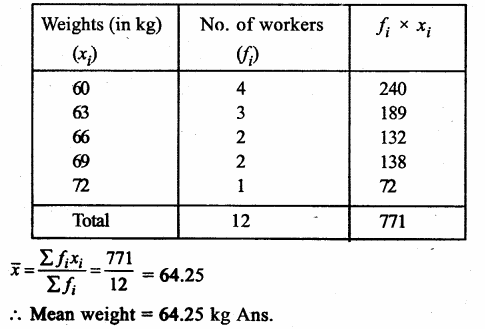Question 3.
Solution: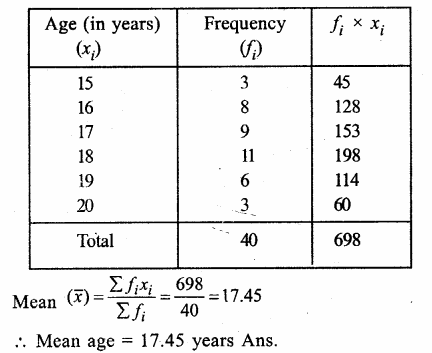Question 4.
Solution: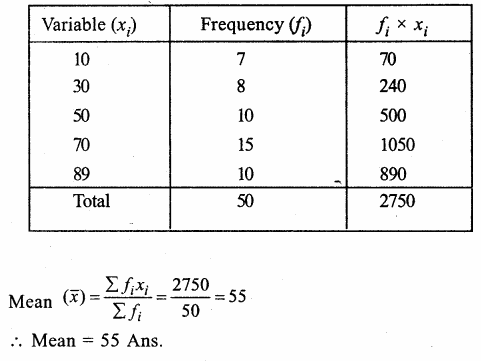Question 5.
Solution:
Mean = 8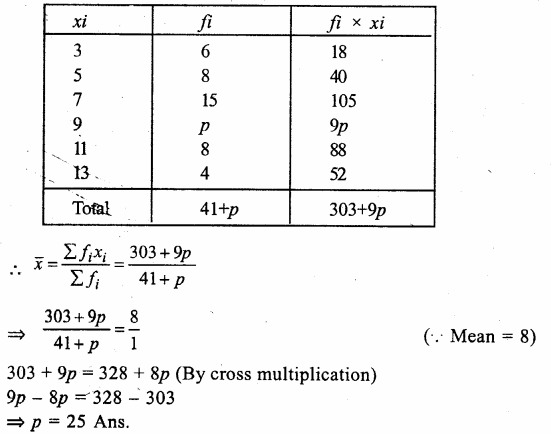Question 6.
Solution:
Mean = 28.25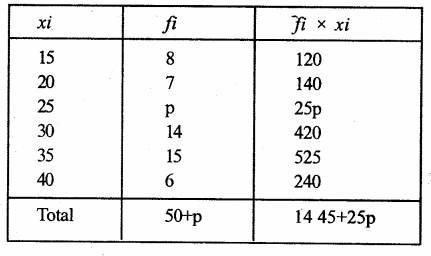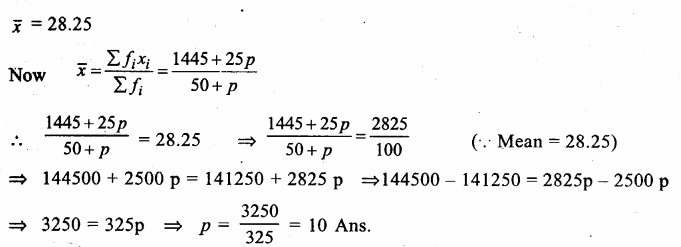Question 7.
Solution:
Mean = 16.6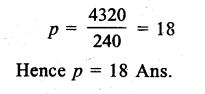Question 8.
Solution:
Mean = 50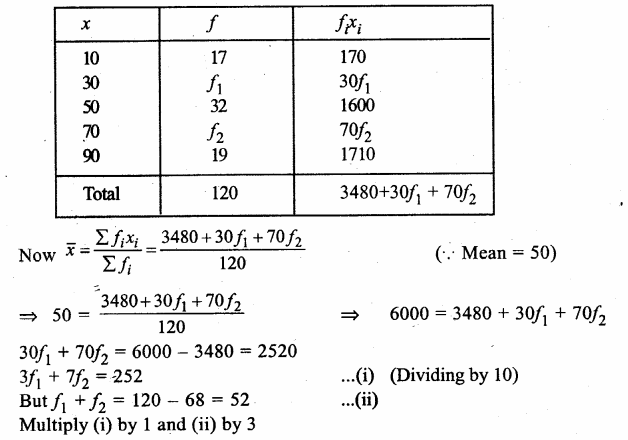Question 9.
Solution: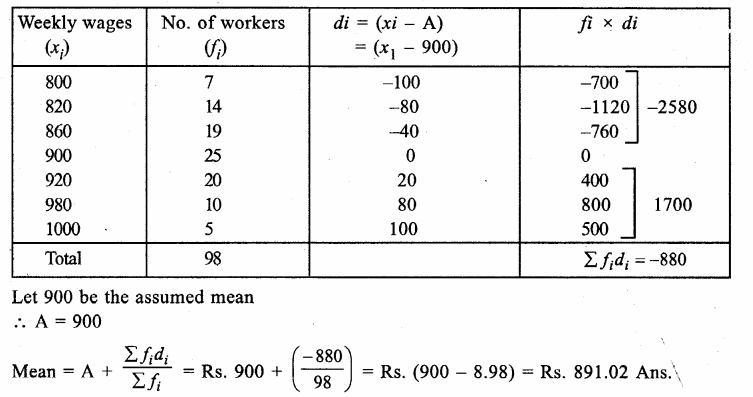Question 10.
Solution:
Let assumed mean = 67Question 11.
Solution:
Here h = 1, Let assumed mean (A) = 21Question 12.
Solution:
Here h = 400 and let assumed mean (A) = 1000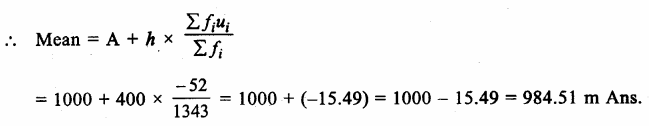Hope given RS Aggarwal Solutions Class 9 Chapter 14 Statistics Ex 14F are helpful to complete your math homework.

If you have any doubts, please comment below. Learn Insta try to provide online math tutoring for you.